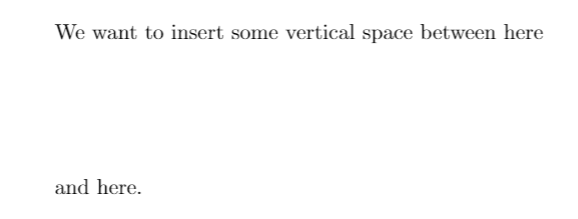This error appears when you have written something inside a count, dimension or skip entry which isn't a number. If you do this, you will generate the error message below:

main.tex, line 5

Missing number, treated as zero.

l<to be read again> t l.8 \vspace{this should be a number} A number should have been here; I inserted 0'. (If you can't figure out why I needed to see a number, look up weird error' in the index to The TeXbook.)

# Common Causes

Forgetting to include a number where a number is required:

The most basic way this error can be generated is if you have forgotten to include a number in a command where a number is required. An example of this is shown below.

We want to insert some vertical space between here

\vspace{this should be a number}

and here.


Here the \vspace{...} command expects a number as its argument, telling it how much vertical space to leave between the text. As there is no number present, an error appears. The correct way to write this

We want to insert some vertical space between here

\vspace{6em}

and here.There are many commands like \vspace{...} which require numbers as their argument. The most common of these are

• Spacing commands such as:
• \vspace{...}
• \vspace*{...}
• \hspace{...}
• \hspace*{...}
• Scaling commands, such as \includegraphics[scale = 0.7]{image}. This will scale your image to be 0.7 times its actual size. The other options available here which require numbers are:
• width
• height
• page
• resolution
• trim
• angle.
• The \linebreak[number] command, where the argument states how many lines you want to be skipped.
• Counters commands such:
• \addtocounter{mycounter}{number}
• \setcounter{mycounter}{number}
• Length setting commands such as \setlenght{\lengthname}{number}. This will change the value of a particular length such as \textwidth to the value number (e.g. \setlength{\textwidth}{1in}).
• Table option commands such as \multicolumn{number}{c}{Table entry}.

Having a linebreak \\ followed by square brackets:

If a linebreak command \\ is ever followed by square brackets [...], it will be taken as an optional argument, and thus a number will be expected inside the square brackets. This is even true if there is whitespace and newlines between the \\ and [...] commands. This problem often appears in tables, as shown below:

\begin{tabular}{c|c}
[x] &  2\\
[x]^2 & 4
\end{tabular}


This will generate an error, as on the third line, we have a linebreak followed by squared brackets. LaTeX expects a number inside the square brackets, but instead finds x. The correct way to write the above table is to include the square brackets inside curly braces {...} as shown below:

\begin{tabular}{c|c}
[x] &  2\\
{[x]}^2 & 4
\end{tabular}


Using the subfigure package:

The subfigure package is long outdated, and will generate a 'Missing number' error when there is no error present. An example of this is shown below:

% In your preamble
\usepackage{subfigure}

% In the body of your document
\begin{figure}
\centering
\begin{subfigure}[b]{0.3\textwidth}
\includegraphics[scale=0.2]{image}
\caption{A gull}
\label{fig:gull}
\end{subfigure}%
\end{figure}


This will generate an error, as the subfigure package does not recognise \textwidth as a number, when it is in fact a number (equivalent to the constant width of the total text block on a page). The way to resolve this is to use the more updated subcaption package, which has replaced subfigure. Writing the same code as above with \usepackage{subcaption} in the preamble instead of \usepackage{subfigure} will give the desired result without any error.